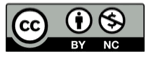# 零基础学习WCDMA(2)：异或

2013-05-24A. 不能
B. 只能用加法
C. 只能用乘法
D. 加法和乘法都可以

## 算法和性质

ABA⊕B

``````A ⊕ A = 假
``````

``````A ⊕ 假 = A
``````

``````(A ⊕ B) ⊕ B = A ⊕ (B ⊕ B) = A ⊕ 假 = A
``````

## (0,1) 模2加法

``````0 + 0 = 0
0 + 1 = 1
1 + 0 = 1
1 + 1 = 0
``````

## (1,–1)乘法

``````1 x 1 = 1
1 x -1 = -1
-1 x 1 = -1
-1 x -1 = 1
``````

``````( 1 -1 1 -1 ) x ( -1 1 -1 1 ) = ( -1 -1 -1 -1 )
``````

## 精彩评论